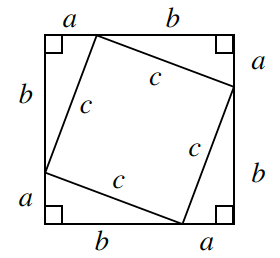Home > CCG > Chapter 7 > Lesson 7.1.3 > Problem7-30

7-30.

Examine the diagram below. Find two equivalent expressions that represent the area of the inner square. Homework Help ✎Area of inner square = (base)(height) or large square minus 4 triangles.

$c^2$ or $a^2+b^2$